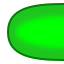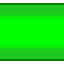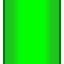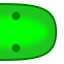Nauč se Python > Materiály > Snake Workshop for PyLadies > Extensions > Snake tile selection

This is a machine-generated translation, meant to support the in-person workshop.

# Picking pieces of a snake #

Instead of having the same piece of snake everywhere, we will always want to choose the right one.

How to do it? According to what should I choose it?

Pictures with pieces of snake are named `from-to`. This is not a coincidence - it shows what you need to know in order to choose the right piece.

When you have a snake according to the following picture, the piece belongs on the tile (3,2) on which the snake crawls from left to up - therefore `snake_tiles['left-top']`.At the ends of the snake, in the names of the pictures, the direction 'end' is replaced.

For each square, you will need to determine where the snake is crawling on it - that is, the direction to the previous and next coordinate.

Coordinate Previous Direction to previous Next Direction to next
(1, 2) not `end` (2, 2) `right`(2, 2) (1, 2) `left` (3, 2) `right`(3, 2) (2, 2) `left` (3, 3) `top`(3, 3) (3, 2) `bottom` (3, 4) `top`(3, 4) (3, 3) `bottom` (3, 5) `top`(3, 5) (3, 4) `bottom` (4, 5) `right`(4, 5) (3, 5) `left` not `end`This is a difficult task. Even though you theoretically know all the necessary information and tools for it now, it is necessary to put them together in the right way. This putting together, algorithm design, is the most complex programming discipline.

Try to think about it, let it settle in your head maybe overnight, go back to the materials from previous lessons (especially the introduction to Python), experiment and discover... And eventually you will figure it out.

If you don't have time, let's see how we can arrange it.

# This is not the bible. #

I am describing one possible solution here. There are many other correct ways to choose the snake's squares. Perhaps you will even find another solution easier - and maybe it will even be your solution!

### Simpler subproblems #

Complicated problems are usually appropriately divided into several simpler problems by programmers. Each one is then solved separately and then put together.

What simpler tasks could be found here?

1. Go through all the coordinates and for each of them, determine the previous and next coordinate of the square.
2. When you have two coordinates, determine the direction from one to the other.

The solution to the first one in the table above is to "fill in" the columns with coordinates. The solution to the second one will give you directions, parts of the image name, from this information.

You will need to solve both problems. However, the second one is easier, so let's focus on it.

### To find out the direction #

You need to tell the computer how to determine the direction from one to the other of two adjacent squares, based on their coordinates.

For example, the direction from (3, 2) to (2, 2) is left. The direction from (3, 2) to (3, 3) is up. (See the picture or the third row of the table above.)In Python, you write it as a function that takes two arguments (coordinates) and returns the English name of the direction - a string that can be used in the file name of the image.

When this function is completed, it should be used as follows:

```>>> direction((3, 2), (2, 2))
'left'
>>> direction((3, 3), (3, 2))
'bottom'
>>> direction((3, 3), 'end')
'end'
```

At the ends of the snake, instead of a pair of numbers, something else will be needed as the second coordinate. The string `'end'` works well, but anything that is not a coordinate could be used, such as `False`, `-1`, or even `[]`. An experienced Pythonista would use the value `None`.

How to write such a function? If you take a closer look at the first three columns of the table above, you may figure out how the coordinates that are to the left of each other differ. Or above each other.

How to determine the direction between two coordinates:

• When the second one is not a coordinate:
• the result is `'end'`
• When the x of the first one equals the second one's x+1:
• the result is `'left'`
• When the x of the first one equals the second one's x-1:
• the result is `'right'`
• When the y of the first one equals the second one's y+1:
• the result is `'bottom'`
• When the y of the first one equals the second one's y-1:
• the result is `'top'`

An experienced programmer will now pay attention and ask: "But what if neither of those conditions is true?" Such a situation may not occur in the game (or maybe it will?), but it is still good to catch it and add, for example, "Otherwise, the result is `'end'`" at the end of the process.

Coming up with this procedure is the more complicated part of solving our problem. The rest is just a nearly literal translation to Python.

```def direction(a, b):
if b == 'end':
return 'end'

# split coordinates to values (a, b)
x_a, y_a = a
x_b, y_b = b

# ... and logic continues
if x_a == x_b + 1:
return 'left'
elif x_a == x_b - 1:
return 'right'
elif y_a == y_b + 1:
return 'bottom'
elif y_a == y_b - 1:
return 'top'
else:
return 'end'

# Try it
print('this should be "left":', direction((3, 2), (2, 2)))
print('this should be "bottom":', direction((3, 3), (3, 2)))
print('this shoudl be "top":', direction((3, 2), (3, 3)))
print('this should be "right":', direction((1, 1), (2, 1)))
print('this should be "end":', direction((3, 3), 'end'))
print('this should be "end":', direction((3, 3), (80, 80)))
```

## Go through all coordinates. #

Now let's go back to the first problem: how to go through all the squares of the snake and find out the previous and next square for each?

Because the difference between coordinates such as (1, 2) and (2, 2) is not very readable at first glance, I will label the pieces of the snake with letters. I will write A instead of (1, 2); B instead of (2, 2); and so on.I will draw such a snake as follows:

• I will draw box A (for which I need to know that it is the beginning and after it is B)
• I will draw box B (for which I need to know that before it is A and after it is B)
• I will draw box C (for which I need to know that before it is B and after it is D)
• ...and so on:
 To draw Previous Next A B C D E F G × A B C D E F B C D E F G ×

How to do it? You already have the first row of the table, the list [A, B, C, D, E, F, G] - these are the coordinates of the snake. When you manage to prepare lists with the second row, [×, A, B, C, D, E, F], and the third one, [B, C, D, E, F, G, ×], you can then combine them using the `zip` function. Do you remember it? It goes through several "conversing" lists and gives a tuple of the first elements, then a tuple of the second elements, then the third...

Our example was:

```Items = ['grass', 'sun', 'carrot', 'river']
Colors = ['green', 'yellow', 'orange', 'blue']
Places = ['on the ground', 'up above', 'on the plate', 'behind the wall']

for item, color, place in zip(items, colors, places):
print(color, item, 'is', place)" in English.
```

But you can use the same way:

```snake = ['A', 'B', 'C', 'D', 'E', 'F', 'G']
prevs = ['x', 'A', 'B', 'C', 'D', 'E', 'F']
nexts = ['B', 'C', 'D', 'E', 'F', 'G', 'x']

for coords, prev, next in zip(snake, prevs, nexts):
print('on the field', coords, 'the snake crawls from', prev, 'to', next)
```

The two additional lists need to be "created" from the first one: select the correct piece and add the "missing" element to the correct side.

```snake = ['A', 'B', 'C', 'D', 'E', 'F', 'G']
prevs = ['end'] + snake[:-1]
nexts = snake[1:] + ['end']

for coords, prev, next in zip(snake, prevs, nexts):
print('on field', coords, 'snake crawls from', prev, 'to', next)
```

Or, with "real" coordinates and the `direction` function:

```snake = [(1, 2), (2, 2), (3, 2), (3, 3), (3, 4), (3, 5), (4, 5)]

for coords, prev, next in zip(snake, ['end'] + snake[:-1], snake[1:] + ['end']):
before = direction(coords, prev)  # direction from current field to previous
after = direction(coords, next)   # direction from current field to next
key = before + '-' + after
print('draw', key, 'at', coords)
```

If you've made it this far, I hope you won't have too much trouble "transplanting" this code into your game.

Toto je stránka lekce z kurzu, který probíhá nebo proběhl naživo s instruktorem.